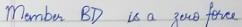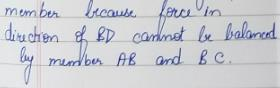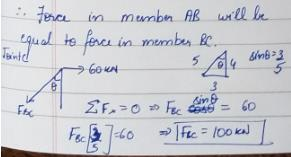Courses

# Test: Trusses -2

## 10 Questions MCQ Test GATE Civil Engineering (CE) 2022 Mock Test Series | Test: Trusses -2

Description
This mock test of Test: Trusses -2 for Civil Engineering (CE) helps you for every Civil Engineering (CE) entrance exam. This contains 10 Multiple Choice Questions for Civil Engineering (CE) Test: Trusses -2 (mcq) to study with solutions a complete question bank. The solved questions answers in this Test: Trusses -2 quiz give you a good mix of easy questions and tough questions. Civil Engineering (CE) students definitely take this Test: Trusses -2 exercise for a better result in the exam. You can find other Test: Trusses -2 extra questions, long questions & short questions for Civil Engineering (CE) on EduRev as well by searching above.
QUESTION: 1

### The force induced in the vertical member CD of the symmetrical plane truss shown in the figure is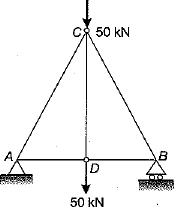Solution:

At join D, the equilibrium shows the force in member CD is 50 kN tensile.

QUESTION: 2

### Consider the following Assumptions in the analysis of a plane truss: 1. The individual members are straight. 2. The individual members are connected by frictionless hinges. 3. The loads and reactions acts only at the joints. Which of the following assumptions are valid?

Solution:

For plane truss assumptions are:
(i) Weight of all members are neglected. All members are straight.
(ii) All connections are smooth, frictionless pins.
(iii) External loads are applied directly to the pin joints.
(iv) All frames are perfect, hence statically determinate.

QUESTION: 3

### A loaded pin-jointed truss is shown in the given figure. The force in member AC is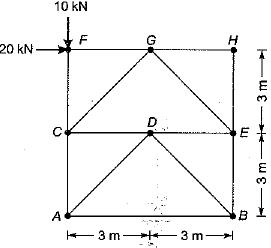Solution:

Cutting a section through members CA, CD, DEand EB and taking moment of the upper part about E.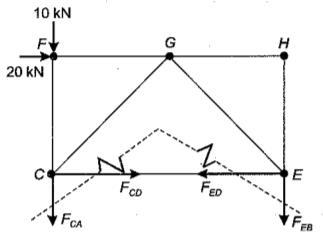∴ Fca x 6 + 10 x 6 - 20 x 3 = 0
∴ Fca = 0

QUESTION: 4

What does the Wiiiiot-Mohr diagram yield?

Solution:
QUESTION: 5

​Which one of the following is the correct statements regarding the force and deflection at point B in trusses I and II shown in the figure?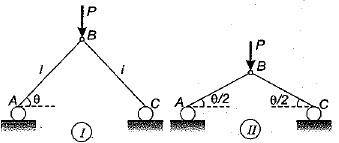Solution:

Force in AB or AC [Consider joint B],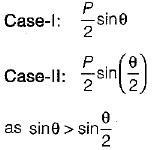So in case I force in members will be less than that in case II.
From symmetry, there will be only vertical deflection of joint B.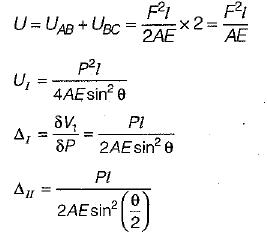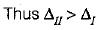QUESTION: 6

A truss ABC carries two horizontal and two vertical loads, as shown in the figure. The horizontal and vertical components of reactions at A will be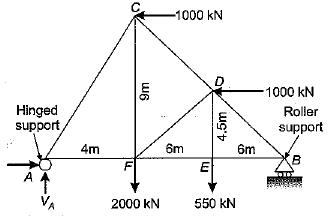Solution:
QUESTION: 7

In the plane truss shown below, how many members have zero force?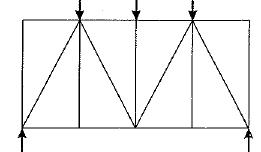Solution:

Six members having zero forces are obvious and seventh member can be from top chord member between the applied loads.

QUESTION: 8

The force in member FD in the given figure is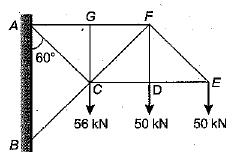Solution:
QUESTION: 9

In the pin-jointed plane truss shown below, what is the magnitude and nature of force in member BC?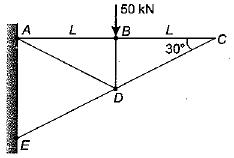Solution:

At joint C there is no external force so force in member BC is zero.

QUESTION: 10

Due to horizontal pull 60 kN at C, what is the force induced in the member AB?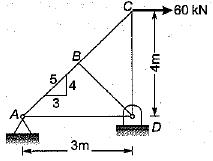Solution: